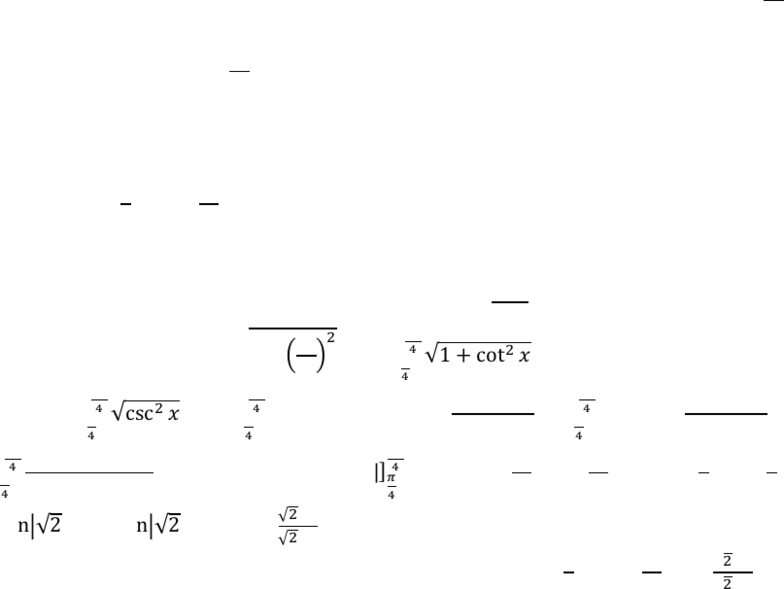Class Notes (1,100,000)
CA (630,000)
UTSG (50,000)
MAT (4,000)
MAT136H1 (900)
all (200)
Lecture

8.1 Arc Length Question #2 (Medium)

Department
Mathematics
Course Code
MAT136H1
Professor
all

Page:
of 18.1 Challenging Integral Applications
Arc Length
Question #2 (Medium): Integral Representation of the Length of the Curve
Strategy:
When the function is given as in terms of , take the derivative of in terms of , thus 
.
If the function is given as in terms of , no need to rearrange to have written in terms of . take the
derivative of it as it is, thus 
, and taking the integral in terms of .
Sample Question:
Set up an integral representing the length of the curve. Then evaluate the exact length of the curve.
  ,

Solution:
First take the derivative of the given function. Thus, 
 . Plug into the formula for the
curve length:  



. Based on the identity  
: 



. multiply by 
: 














Therefore, the length of the curve    over the interval

is 
